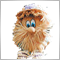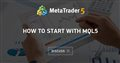# Custom Indicator in EA not working20

Hi,

When I apply my indicator to a regular chart it works properly, while when I use it in EA it returns always 0 value (as if it was not calculated).

I call indicator using: iCustom(NULL, 0, "my_indicator", 0, 1);

I have put Print commands to Init and OnCalculate of the indicator and they only print when EA is paused.

Any ideas?

Thanks,

T.Moderator
215966

tomasz.maciejak :

Hi,

When I apply my indicator to a regular chart it works properly, while when I use it in EA it returns always 0 value (as if it was not calculated).

I call indicator using: iCustom(NULL, 0, "my_indicator", 0, 1);

I have put Print commands to Init and OnCalculate of the indicator and they only print when EA is paused.

Any ideas?

Thanks,

T.

Where is your code? We do not know how to read other people's thoughts - we understand only if there is a code.Moderator
215966

tomasz.maciejak :

Hi,

When I apply my indicator to a regular chart it works properly, while when I use it in EA it returns always 0 value (as if it was not calculated).

I call indicator using: iCustom(NULL, 0, "my_indicator", 0, 1);

I have put Print commands to Init and OnCalculate of the indicator and they only print when EA is paused.

Any ideas?

Thanks,

T.

An example of working with iCustom: An example of working with iCustom - we get the indicator data in the EA [data folder]\MQL5\Indicators\Examples\MACD.mq5• 2020.09.06
• www.mql5.com
This thread discusses MQL5 code examples. There will be examples of how to get data from indicators, how to program advisors...20

Hi,

The point is why it works on regular chart but it does not on EA. I attach the OnCalculate (onInit only prepares TimeSeries and sets them to 0).

The procedure should calculate minimums and maximum of waves and their cumulative volume. Wave is increasing closes or decreasing closes with some tolerance.

```nt OnCalculate(const int rates_total,
const int prev_calculated,
const datetime &time[],
const double &open[],
const double &high[],
const double &low[],
const double &close[],
const long &tick_volume[],
const long &volume[],
{
int cnt_periods_back=rates_total-prev_calculated-1;

if (cnt_periods_back<0)
return rates_total;

cumulativeVolume1[cnt_periods_back]=(double)Volume[cnt_periods_back];

last_min[cnt_periods_back]=Close[cnt_periods_back];
last_max[cnt_periods_back]=Close[cnt_periods_back];

for (int i=cnt_periods_back-1;i>0;i--) {
if (Close[i+1]<Close[i] && wavetrend1[i+1]!=1) {
//local minimum
if (deviation_ref==0 && Close[i]-Close[i+1] < pips_tolerance*Point) {
deviation_ref=Close[i+1];
cumulativeVolume1[i]=cumulativeVolume1[i+1]+Volume[i];
wavetrend1[i]=-1;
last_min[i]=last_min[i+1];
last_max[i]=last_max[i+1];

continue;
}
if (deviation_ref>0 && Close[i]-deviation_ref < pips_tolerance*Point) {

cumulativeVolume1[i]=cumulativeVolume1[i+1]+Volume[i];
wavetrend1[i]=-1;
last_min[i]=last_min[i+1];
last_max[i]=last_max[i+1];
continue;
}

wavetrend1[i]=1;
cumulativeVolume1[i]=(double)Volume[i];
last_min[i]=Close[i+1];
lev1waves_mins[lev1waves_mins_cnt].time=Time[i+1];
lev1waves_mins[lev1waves_mins_cnt].extremum=Close[i+1];
lev1waves_mins[lev1waves_mins_cnt].cumulativeVolume=(long)cumulativeVolume1[i+1];
lev1waves_mins_cnt++;
last_max[i]=last_max[i+1];
if (lev1waves_mins_cnt==ArraySize(lev1waves_mins)-1)
if (-1==ArrayResize(lev1waves_mins, ArraySize(lev1waves_mins)*2, 0))
Print("Min not resized");
}
else
if (Close[i+1]>Close[i] && wavetrend1[i+1]!=-1) { // change of trend - new maximum in i+1

//down trend - change of trend
if (deviation_ref==0 && Close[i+1]-Close[i] < pips_tolerance*Point) {
deviation_ref=Close[i+1];
cumulativeVolume1[i]=cumulativeVolume1[i+1]+Volume[i];
wavetrend1[i]=1;
last_min[i]=last_min[i+1];
last_max[i]=last_max[i+1];

continue;
}
if (deviation_ref>0 && deviation_ref-Close[i] < pips_tolerance*Point) {

cumulativeVolume1[i]=cumulativeVolume1[i+1]+Volume[i];
wavetrend1[i]=1;
last_min[i]=last_min[i+1];
last_max[i]=last_max[i+1];

continue;
}
wavetrend1[i]=-1;
cumulativeVolume1[i]=(double)Volume[i];
last_max[i]=Close[i+1];
lev1waves_maxs[lev1waves_maxs_cnt].time=Time[i+1];
lev1waves_maxs[lev1waves_maxs_cnt].extremum=Close[i+1];
lev1waves_maxs[lev1waves_maxs_cnt].cumulativeVolume=(long)cumulativeVolume1[i+1];

if (lev1waves_maxs_cnt==ArraySize(lev1waves_maxs)-1)
if (-1==ArrayResize(lev1waves_maxs, ArraySize(lev1waves_maxs)*2, 0))
Print("Max not resized");

lev1waves_maxs_cnt++;
last_min[i]=last_min[i+1];
}
else
{

cumulativeVolume1[i]=cumulativeVolume1[i+1]+Volume[i];
wavetrend1[i]=wavetrend1[i+1];
last_min[i]=last_min[i+1];
last_max[i]=last_max[i+1];
if (deviation_ref>0) {
if (wavetrend1[i]==1 && Close[i]>deviation_ref)
deviation_ref=0;
else
if (wavetrend1[i]==-1 && Close[i]<deviation_ref)
deviation_ref=0;
}

}
}//for loop
return(rates_total);
}

```Moderator
215966

tomasz.maciejak :

Hi,

The point is why it works on regular chart but it does not on EA. I attach the OnCalculate (onInit only prepares TimeSeries and sets them to 0).

The procedure should calculate minimums and maximum of waves and their cumulative volume. Wave is increasing closes or decreasing closes with some tolerance.

Have you run my example?20

Have you run my example?

Your example is for MQL5. I write in MQL4. Concept of iCustom has changed. In MQL4 iCustom returned value, in MQL5 it returns handle. So it won't work for me.Moderator
215966

tomasz.maciejak :
Your example is for MQL5. I write in MQL4. Concept of iCustom has changed. In MQL4 iCustom returned value, in MQL5 it returns handle. So it won't work for me.

You are on the MQL5 forum. Naturally, you received an answer to MQL5. If you have a question about an old terminal - ask it in a special section ( MQL4 and MetaTrader 4 ) - this way you will not waste time from users.

I will move this topic to the section of the old terminal.23254

1. Why did you post your MT4 question in one of the MT5 sections instead of the MQL4 section, (bottom of the Root page?)
General rules and best pratices of the Forum. - General - MQL5 programming forum?
Next time post in the correct place. A moderator has already moved this thread to the proper place.

2. tomasz.maciejak: The point is why it works on regular chart but it does not on EA. I attach the OnCalculate  (onInit only prepares TimeSeries and sets them to 0).
3. OnInit shouldn't be doing that. It should be creating buffers out of your empty arrays. Not initializing them. Post your code.

4. You attached the OnCalculate which is useless since you say it work when attached to a chart. Your call in the EA uses buffer index zero. We can't possibly know if that is correct since you haven't posted your code (№ 3).

`iCustom(NULL, 0, "my_indicator", 0, 1);`20

William Roeder:
1. Why did you post your MT4 question in one of the MT5 sections instead of the MQL4 section, (bottom of the Root page?)
General rules and best pratices of the Forum. - General - MQL5 programming forum?
Next time post in the correct place. A moderator has already moved this thread to the proper place.

2. OnInit shouldn't be doing that. It should be creating buffers out of your empty arrays. Not initializing them. Post your code.

3. You attached the OnCalculate which is useless since you say it work when attached to a chart. Your call in the EA uses buffer index zero. We can't possibly know if that is correct since you haven't posted your code (№ 3).

Thank you for prompt response.

1. By mistake. Sorry. It's my first post here.

3. OnInit - by initialization I meant SetIndexEmptyValue function. Code below:

```   current_chart_id=ChartID();
IndicatorDigits(0);

SetIndexBuffer(0,wavetrend1);
SetIndexLabel(0, NULL);
SetIndexEmptyValue(0, 0.0);

SetIndexBuffer(1,cumulativeVolume1);
SetIndexLabel(1, NULL);
SetIndexEmptyValue(1, 0.0);

SetIndexBuffer(2,last_min);
SetIndexLabel(2, NULL);
SetIndexEmptyValue(2, 0.0);

SetIndexBuffer(3,last_max);
SetIndexLabel(3, NULL);
SetIndexEmptyValue(5, 0.0);

seq=0;
deviation_ref=0;
lev1waves_mins_cnt=0;
lev1waves_maxs_cnt=0;
return(INIT_SUCCEEDED);```20

I solved it myself. The called indicator had a bug which ended in exception, but the exception was not visible on any log (extensive debugging helped)